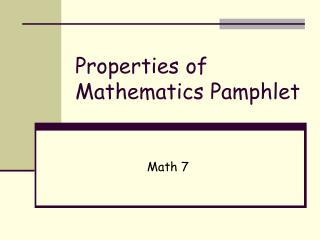DownloadDownload PresentationProperties of Mathematics Pamphlet

# Properties of Mathematics Pamphlet

Télécharger la présentation## Properties of Mathematics Pamphlet

- - - - - - - - - - - - - - - - - - - - - - - - - - - E N D - - - - - - - - - - - - - - - - - - - - - - - - - - -
##### Presentation Transcript

1. Name of the Property • In words:

2. Multiplicative Property of Zero • In words: Any number multiplied by zero is zero.

3. Multiplicative Property of Zero • In words: Any number multiplied by zero is zero.

4. Identity Property of Multiplication • In words: Any number multiplied by one is equal to itself.

5. Identity Property of Multiplication • In words: Any number multiplied by one is equal to itself.

6. Identity Property of Addition • In words: Any number plus zero is equal to itself.

7. Identity Property of Addition • In words: Any number plus zero is equal to itself.

8. Commutative Property of Addition • In words: If you change the order of the numbers you are adding, the answer is the same.

9. Commutative Property of Addition • In words: If you change the order of the numbers you are adding, the answer is the same.

10. Commutative Property of Multiplication • In words: If you change the order of the numbers you are multiplying, the answer is the same.

11. Commutative Property of Multiplication • In words: If you change the order of the numbers you are multiplying, the answer is the same.

12. Associative Property of Addition • In words: If you change the location of the parenthesis in an addition problem, the answer is the same.

13. Associative Property of Addition • In words: If you change the location of the parenthesis in an addition problem, the answer is the same.

14. Associative Property of Multiplication • In words: If you change the location of the parenthesis in a multiplication problem, the answer is the same.

15. Associative Property of Multiplication • In words: If you change the location of the parenthesis in a multiplication problem, the answer is the same.

16. Test Yourself • Which property is demonstrated by the following statement?

17. Test Yourself • Which property is demonstrated by the following statement?

18. Test Yourself • Which property is demonstrated by the following statement?

19. Test Yourself • Which property is demonstrated by the following statement?

20. Test Yourself • Which property is demonstrated by the following statement?

21. Test Yourself • Which property is demonstrated by the following statement?

22. Test Yourself • Which property is demonstrated by the following statement?